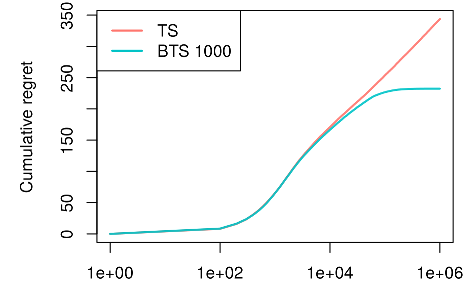library(contextual)

# Replication of THOMPSON SAMPLING WITH THE ONLINE BOOTSTRAP By Dean Eckles and Maurits Kaptein

# This evaluations takes time - up to a few hours when run single core.

# Running the script in parallel (for example, on 8 cores)
# shortens the evaluation time substantially.

# https://arxiv.org/abs/1410.4009

# Fig 2. Empirical regret for Thompson sampling and BTS in a K-armed binomial bandit problem.

bandit             <- BasicBernoulliBandit$new(weights = c(0.5, rep(0.4,9))) agents <- list(Agent$new(BootstrapTSPolicy$new(1000), bandit, "BTS 1000"), Agent$new(ThompsonSamplingPolicy$new(), bandit, "TS")) simulator <- Simulator$new(agents        = agents,
do_parallel   = TRUE,
save_interval = 50,
set_seed      = 999,
horizon       = 1e+05,
simulations   = 1000)

simulator$run() plot(simulator$history, log = "x")